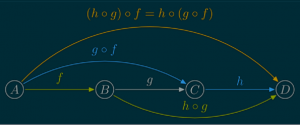# August 2013

You are currently browsing the monthly archive for August 2013.

## Yann Esposito’s Category theory Slides with Haskell

I really enjoyed Yann Esposito’s slides “Category Theory Presentation” which give a relatively simple and artistic introduction to category theory for Haskell programmers.  (I like Haskell too.)  His slides present the basic definitions of categories, functors, natural transformations, and monads along with working Haskell code.

Here is a fragment of a typical slide.## Category Theory ?

Artificial intelligence and machine learning are linked with a huge list of other fields of study: statistics, probability, information theory, computational complexity theory, algorithmic complexity theory, linear regression, linear programming, approximation theory, neuroscience, automata theory, geometry of high dimensional spaces, reproducing kernal Hilbert spaces, optimization, formal languages, and many other areas (see e.g. the graphic below by Swami Chandrasekaran).

My focus has always been on applied mathematics rather than “pure” mathematics. But, by studying AI I was almost forced into learning about areas that I had avoided in graduate school like decidability (logic) and proof theory which did not seem practical at the time.  I felt that most abstract mathematics and some abstract computer science, though beautiful, were not very useful. So, I am surprised that I am now studying the most abstract and possibly most useless area of mathematics, Category Theory.

Why category theory (aka “Abstract Nonsense“)?  Mathematicians often wonder “What is the simplest example of this idea?”, “Where do idea A and idea B intersect?”, and “How does this idea generalize?”.  Category theory seems to be about theses questions which I hope will be useful for AI research.  I was also inspired to read about category theory by Ireson-Paine‘s article “What Might Category Theory do for Artificial Intelligence and Cognitive Science?” and “Ologs: A Categorical Framework for Knowledge Representation” by Spivak and Kent (2012). Paine describes relationships between category theory, logical unification, holographic reduced representation, neural nets, the Physical Symbol System Hypothesis, analogical reasoning, and logic. The article includes many authoritative interesting references and links.  Ologs appear to be a category theoretic version of semantic networks.

Since I am studying category theory in my off time, I will probably blog about it. I am an enthusiastic beginner when it comes to category theory, so hopefully I can communicate that enthusiasm and avoid posting the common conceptual blunders that are part of learning a new field.

Cheers, Hein

PS:  I loved this graphic by Swami Chandrasekaran from the article “Becoming a Data Scientist“.Road To Data Science by Swami Chandrasekaran

## The Exact Standard Deviation of the Sample Median

In a previous post, I gave the well-known approximation to the standard deviation of the sample median

$$\sigma \approx {1 \over 2\sqrt{n}\,f(x_m)}$$

where $f(x)$ is the probability density function and $x_m$ is the median (see Laplace and Kenney and Keeping).  Here are some examples.

 Distribution Median Approx StD of Median Standard Gaussain mean 0 std 1 0 $\sqrt{\pi \over{2 n}}$ Uniform 0 to 1 1/2 $1\over{2\sqrt{n}}$ Logistic with mean 0 and shape $\beta$ 0 ${2\beta}\over{\sqrt{n}}$ Student T with mean 0 and $\nu$ deg free 0 $\frac{\sqrt{\nu }\ B\left(\frac{\nu }{2},\frac{1}{2}\right)}{2 \sqrt{n}}$

$\$

Computing the exact standard deviation of the sample median is more difficult. You first need to find the probability density function of the sample median which is

$$f_m(x) = g(c(x)) f(x)$$

where

$$g(x) = \frac{(1-x)^{\frac{n-1}{2}} x^{\frac{n-1}{2}}}{B\left(\frac{n+1}{2},\frac{n+1}{2}\right)},$$
$B$ is the beta function, $c(x)$ is the cumulative distribution function of the sample distribution, and $f(x)$ is the probability density function of the sample distribution.

Now the expected value of the sample median is

$$\mu_m = \int x f_m(x) dx$$

and the standard deviation of the sample median is

$$\sigma_m = \sqrt{\int (x-\mu_m)^2 f_m(x)\ dx}.$$

Generally speaking, these integrals are hard, but they are fairly simple for the uniform distribution.  If the sample distribution is uniform between 0 and 1, then

$f(x) = 1,$

$c(x) = x,$

$g(x) = \frac{(1-x)^{\frac{n-1}{2}} x^{\frac{n-1}{2}}}{B\left(\frac{n+1}{2},\frac{n+1}{2}\right)},$

$f_m(x) = g(x),$

$\mu_m = \int x g(x) dx \ =\ 1/2,$ and

$$\sigma_m = \sqrt{\int (x-\mu_m)^2 f_m(x)\ dx}\ = \ {1\over{2\sqrt{n+2}}}$$

which is close to the approximation given in the table.

(Technical note:  The formulas above only apply for odd values of $n$ and continuous sample probability distributions.)

If you want the standard deviation for the sample median of a particular distribution and a $n$, then you can use numerical integration to get the answer. If you like, I could compute it for you.  Just leave a comment indicating the distribution and $n$.

I wonder if there is some way to identify which internet queries are the most frequently asked and least frequently successfully answered.

I bring this up because my three most popular blog posts are: